# Bond Grindability Test Procedure

The Bond Ball Mill Grindability test is designed to give a measure of the grinding characteristics of a material by finding the amount of size reduction resulting from a controlled energy input.

Ball Mill Grindability of a material depends upon its hardness, structure, the manner in which it is fractured. The grindability is useful in evaluating power requirements for grinding. To design a particle breakage system it is important to know the energy required to achieve a given size reduction. The most commonly used energy-size relationship is Bond’s third law equation.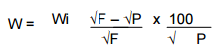Wi = work index of the material comminuted.  It is a relative measure of the resistance of the material to fracture.
F, P = feed and product 80% passing size in microns
W = energy required, kWh/short ton.
Useful forms of this equation are as follows: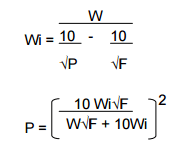For the ball mill grindability test, the work index is calculated from the following revised equation (in short tons):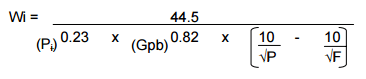where:
Pi = sieve size tested (microns)
Gpb = ball mill grind ability
Wi, P & F have the same meanings as in the top equation.

Equipment required:

Laboratory ball mill, 34.02 cm x 34.02 cm with following ball charge: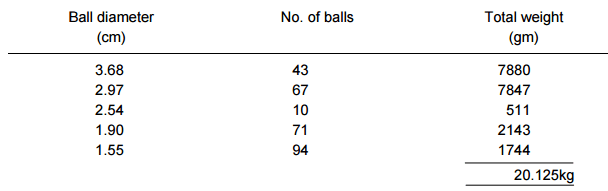• Agitator rolls.
• Measuring cylinder, 700ml volume.
• Set of screens, including the following sizes:
850µm, 600µm, 425µm, 300µm, 210µm, 150µm
• Ro-tap machine, with timer.
• Drying oven.
• Pressure filter

Sample Preparation

1. Obtain about 5 – 6 kg of material to be tested and dry thoroughly.
2. Crush dried material minus 3360µm.
3. Thoroughly blend the crushed product and then split out a sample for screen analysis.

Procedures

1. Carry out wet and dry sizing on the split sample. The percentage of under-size from the screen which is the required size (297µm in this case) is the percentage of finished material in the feed.
2. Fill the 700ml test can with ore and compact by shaking. Add more ore as necessary until further compaction ceases.
3. Weight and transfer ore to the ball mill.
4. Grind dry for 100 revolutions.
5. Empty the ball charge and ore through a coarse screen to separate the balls from the ore.
6. After mill and balls are swept clean, screen and ground ore through the mesh screen to be tested, using coarser protective screens if necessary. (Note: due to the large sample size, it will probably be necessary to screen the sample in a number of smaller portions.)
7. Determine and record:
weight oversize;
weight undersize;
corrected undersize
(assume weight differences are
associated with undersize only)
8. Add to the oversize a quantity of new unsegregated feed equal to the weight of corrected under-size.
9. Return the oversize and new feed to the ball mill.
10. Calculate the number of revolutions to produce a circulating load of 250%.
11. Allow the mill to rotate the required number of times.
12. Repeat steps 7 through 11 until the net grams of sieve undersize produced per mill revolution reaches equilibrium and reverses its direction of increase or decrease.
13. Screen entire mill charge through required mesh screen. Do screen analysis on oversize and undersize.
14. Plot cumulative weight percent passing VS particle size (in microns) for feed, final cycle oversize and final cycle undersize.
15. Determine d80 for feed and last cycle undersize (d80 is the screen opening in microns through which 80% material passes).
16. Calculate average grams of undersize produced per revolution for the last three cycles (Gpb).
17. Calculate work index using the second equation.where:
X = number of revolutions required.
W = weight of original feed.
Y = corrected weight undersize previous cycle.
P = percent undersize in original feed.
R = revolutions previous cycle.
U = actual undersize produced previous cycle.

Determination of d80 values:  d80 = X + Y (Z – X)

where:
d80 = 80% passing size.
X = micron size of screen through which nearest percentage below 80% passes.
Z = micron size of screen through which nearest percentage above 80% passes.
Y = ratio of the difference between 80% and the nearest cumulative percent below 80% AND the difference between the nearest cumulative percent above 80% and the nearest cumulative percent below 80%.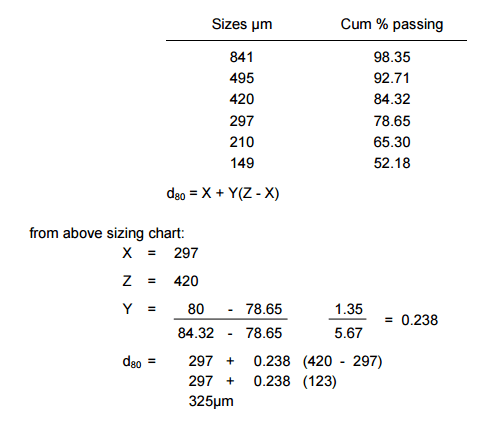Ball Mill Grindability (Gpb) = (gm/rev) average of last three grinding cycles to give 250% circulating load.

Precautions: There should be consistency in compaction of ores in the 700ml test can. This is normally done by shaking. Accurate observations on grinding revolutions are essential. Dry screening procedures should be consistent. Gpb calculations should aim for maximum accuracy.